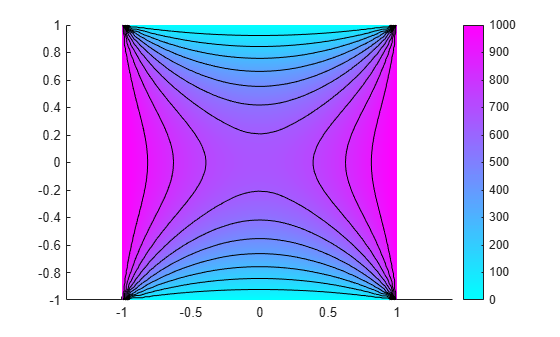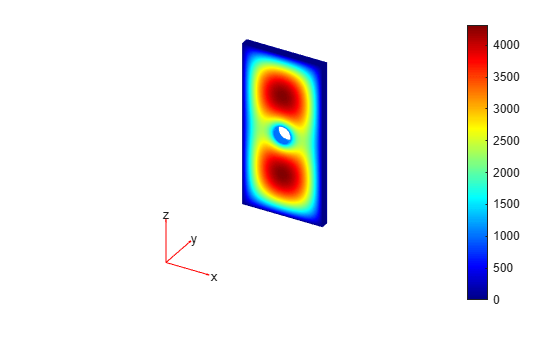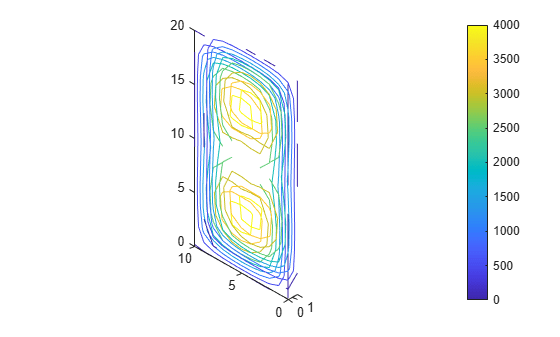# interpolateElectricPotential

Interpolate electric potential in electrostatic or DC conduction result at arbitrary spatial locations

Since R2021a

## Syntax

``Vintrp = interpolateElectricPotential(results,xq,yq)``
``Vintrp = interpolateElectricPotential(results,xq,yq,zq)``
``Vintrp = interpolateElectricPotential(results,querypoints)``

## Description

example

````Vintrp = interpolateElectricPotential(results,xq,yq)` returns the interpolated electric potential values at the 2-D points specified in `xq` and `yq`.```

example

````Vintrp = interpolateElectricPotential(results,xq,yq,zq)` uses 3-D points specified in `xq`, `yq`, and `zq`.```

example

````Vintrp = interpolateElectricPotential(results,querypoints)` returns the interpolated electric potential values at the points specified in `querypoints`.```

## Examples

collapse all

Create an electromagnetic model for electrostatic analysis.

`emagmodel = createpde("electromagnetic","electrostatic");`

Create a square geometry and include it in the model. Plot the geometry with the edge labels.

```R1 = [3,4,-1,1,1,-1,1,1,-1,-1]'; g = decsg(R1, 'R1', ('R1')'); geometryFromEdges(emagmodel,g); pdegplot(emagmodel,"EdgeLabels","on") xlim([-1.5 1.5]) axis equal```Specify the vacuum permittivity in the SI system of units.

`emagmodel.VacuumPermittivity = 8.8541878128E-12;`

Specify the relative permittivity of the material.

`electromagneticProperties(emagmodel,"RelativePermittivity",1);`

Apply the voltage boundary conditions on the edges of the square.

```electromagneticBC(emagmodel,"Voltage",0,"Edge",[1 3]); electromagneticBC(emagmodel,"Voltage",1000,"Edge",[2 4]);```

Specify the charge density for the entire geometry.

`electromagneticSource(emagmodel,"ChargeDensity",5E-9);`

Generate the mesh.

`generateMesh(emagmodel);`

Solve the model and plot the electric potential.

```R = solve(emagmodel); pdeplot(emagmodel,"XYData",R.ElectricPotential, ... "Contour","on") axis equal```Interpolate the resulting electric potential to a grid covering the central portion of the geometry, for `x` and `y` from `-0.5` to `0.5`.

```v = linspace(-0.5,0.5,51); [X,Y] = meshgrid(v); Vintrp = interpolateElectricPotential(R,X,Y)```
```Vintrp = 2601×1 602.2959 616.0368 629.0799 641.4304 653.0883 664.0538 674.4221 684.1447 693.2593 701.7856 ⋮ ```

Reshape `Vintrp` and plot the resulting electric potential.

```Vintrp = reshape(Vintrp,size(X)); figure contourf(X,Y,Vintrp) colormap(cool) colorbar```Alternatively, you can specify the grid by using a matrix of query points.

```querypoints = [X(:),Y(:)]'; Vintrp = interpolateElectricPotential(R,querypoints);```

Create an electromagnetic model for electrostatic analysis.

`emagmodel = createpde("electromagnetic","electrostatic");`

Import and plot the geometry representing a plate with a hole.

```importGeometry(emagmodel,"PlateHoleSolid.stl"); pdegplot(emagmodel,"FaceLabels","on","FaceAlpha",0.3)```Specify the vacuum permittivity in the SI system of units.

`emagmodel.VacuumPermittivity = 8.8541878128E-12;`

Specify the relative permittivity of the material.

`electromagneticProperties(emagmodel,"RelativePermittivity",1);`

Specify the charge density for the entire geometry.

`electromagneticSource(emagmodel,"ChargeDensity",5E-9);`

Apply the voltage boundary conditions on the side faces and the face bordering the hole.

```electromagneticBC(emagmodel,"Voltage",0,"Face",3:6); electromagneticBC(emagmodel,"Voltage",1000,"Face",7);```

Generate the mesh.

`generateMesh(emagmodel);`

Solve the model.

`R = solve(emagmodel)`
```R = ElectrostaticResults with properties: ElectricPotential: [4919x1 double] ElectricField: [1x1 FEStruct] ElectricFluxDensity: [1x1 FEStruct] Mesh: [1x1 FEMesh] ```

Plot the electric potential.

`pdeplot3D(emagmodel,"ColorMapData",R.ElectricPotential)`Interpolate the resulting electric potential to a grid covering the entire geometry, for `x`, `y`, and `z`.

```x = linspace(0,10,11); y = linspace(0,1,5); z = linspace(0,20,11); [X,Y,Z] = meshgrid(x,y,z); Vintrp = interpolateElectricPotential(R,X,Y,Z);```

Reshape `Vintrp`.

`Vintrp = reshape(Vintrp,size(X));`

Plot the resulting electric potential as a contour slice plot for two values of the y-coordinate.

```figure contourslice(X,Y,Z,Vintrp,[],[0 1],[]) view([10,10,-10]) axis equal colorbar```## Input Arguments

collapse all

Solution of an electrostatic or DC conduction problem, specified as an `ElectrostaticResults` or `ConductionResults` object. Create `results` using the `solve` function.

Example: `results = solve(emagmodel)`

x-coordinate query points, specified as a real array. `interpolateElectricPotential` evaluates the electric potential at the 2-D coordinate points `[xq(i) yq(i)]` or at the 3-D coordinate points `[xq(i) yq(i) zq(i)]` for every `i`. Because of this, `xq`, `yq`, and (if present) `zq` must have the same number of entries.

`interpolateElectricPotential` converts the query points to column vectors `xq(:)`, `yq(:)`, and (if present) `zq(:)`. It returns electric potential values as a column vector of the same size. To ensure that the dimensions of the returned solution are consistent with the dimensions of the original query points, use `reshape`. For example, use `Vintrp = reshape(Vintrp,size(xq))`.

Example: `xq = [0.5 0.5 0.75 0.75]`

Data Types: `double`

y-coordinate query points, specified as a real array. `interpolateElectricPotential` evaluates the electric potential at the 2-D coordinate points `[xq(i) yq(i)]` or at the 3-D coordinate points `[xq(i) yq(i) zq(i)]` for every `i`. Because of this, `xq`, `yq`, and (if present) `zq` must have the same number of entries.

`interpolateElectricPotential` converts the query points to column vectors `xq(:)`, `yq(:)`, and (if present) `zq(:)`. It returns electric potential values as a column vector of the same size. To ensure that the dimensions of the returned solution are consistent with the dimensions of the original query points, use `reshape`. For example, use `Vintrp = reshape(Vintrp,size(yq))`.

Example: `yq = [1 2 0 0.5]`

Data Types: `double`

z-coordinate query points, specified as a real array. `interpolateElectricPotential` evaluates the electric potential at the 3-D coordinate points `[xq(i) yq(i) zq(i)]`. Therefore, `xq`, `yq`, and `zq` must have the same number of entries.

`interpolateElectricPotential` converts the query points to column vectors `xq(:)`, `yq(:)`, and `zq(:)`. It returns electric potential values as a column vector of the same size. To ensure that the dimensions of the returned solution are consistent with the dimensions of the original query points, use `reshape`. For example, use `Vintrp = reshape(Vintrp,size(zq))`.

Example: `zq = [1 1 0 1.5]`

Data Types: `double`

Query points, specified as a real matrix with either two rows for 2-D geometry or three rows for 3-D geometry. `interpolateElectricPotential` evaluates the electric potential at the coordinate points `querypoints(:,i)` for every `i`, so each column of `querypoints` contains exactly one 2-D or 3-D query point.

Example: For a 2-D geometry, ```querypoints = [0.5 0.5 0.75 0.75; 1 2 0 0.5]```

Data Types: `double`

## Output Arguments

collapse all

Electric potential at query points, returned as a vector. For query points that are outside the geometry, `Vintrp(i)` = `NaN`.

## Version History

Introduced in R2021a

expand all